# Excercise 5.2 Lines-and-Angles - NCERT Solutions Class 7

Go back to  'Lines and Angles'

## Chapter 5 Ex.5.2 Question 1

State the property that is used in each of the following statements?

(i)   If $$a || b,$$ then $$\angle1 = \angle5.$$

(ii)  If $$\angle4 = \angle6,$$ then $$a || b.$$

(iii) If $$\angle4 + \angle5 = 180^\circ,$$ then $$a || b.$$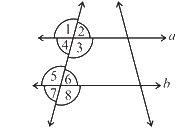### Solution

Steps:

(i) If two parallel lines are intersected by a transversal, then each pair of corresponding angles are equal .

(ii) When a transversal intersects two parallel lines such that if pair of alternate interior angles are equal then the lines are parallel.

(iii) When a transversal intersects two parallel line such that pair of interior angles on the same side of transversal are supplementary, then the lines are parallel.

## Chapter 5 Ex.5.2 Question 2

(i) The pairs of corresponding angles.

(ii) The pairs of alternate interior angles.

(iii) Pairs of interior angles on the same side of the transversal.

(iv) The vertically opposite angles.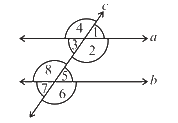### Solution

Steps:

(i) $$\angle1$$ and $$\angle5$$; and $$\angle2$$ and $$\angle6$$;$$\angle4$$ and $$\angle8$$;$$\angle3$$ and $$\angle7$$;

(ii) $$\angle3\rm \,and\,\angle5 \,, \angle2 \,and\,\angle8$$

(iii) $$\angle3\,{\text {and} }\,\angle8,\angle2\,{\text {and} }\,\angle5$$

(iv) $$\angle1$$ and $$\angle3$$$$\angle2$$ and $$\angle4$$$$\angle6$$ and $$\angle8$$$$\angle5$$ and $$\angle7$$

## Chapter 5 Ex.5.2 Question 3

In the adjoining figure, $$p || q$$. Find the unknown angles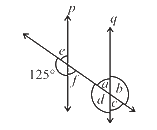### Solution

Reasoning:

First, by using linear pair find the measure of $$\angle e$$ and then find the corresponding and vertically opposite angle to $$\angle e$$ and again by using linear pair find the value of $$\angle b$$ and its vertically opposite angle.

Steps:

Given $$p || q$$ and it is intersected by a transversal.

$$\angle = 125^\circ\\ \text{(Corresponding angle)}$$

Since,

$$125^\circ+ \angle e = 180^\circ \\ \text{(Linear pair)}$$

$$\angle e = 180^\circ - 125^\circ$$

$$\angle e = 55^\circ$$

$$\angle e = \angle f = 55^\circ \\ \text{(Vertically opposite angles)}$$

$$\angle e = \angle a = 55^\circ \\ \text{(Corresponding angles)}$$

$$\angle a + \angle b = 180^\circ \\ \text{ (Linear pair)}$$

$$55^\circ + \angle b = 180^\circ$$

$$\angle b = 180^\circ - 55^\circ$$

$$\angle b =125^\circ$$

Also,

$$\angle b = \angle d = 125^\circ \\ \text{(Vertically opposite angles)}$$

$$\angle a = \angle c = 55^\circ \\ \text{(Vertically opposite angles)}$$

Thus,

$$\angle a = 55^\circ; \angle b = 125^\circ; \angle c = 55^\circ;$$

$$\angle d = 125^\circ;\angle e = 55^\circ ; \angle f = 55^\circ$$

## Chapter 5 Ex.5.2 Question 4

Find the value of $$x$$ in each of the following figures if  $$l || m.$$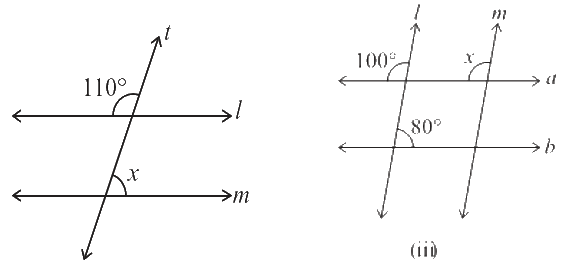### Solution

(i) Reasoning:

There are two operations done in sequence. First, find the corresponding angle to $$110^\circ$$ i. e $$\angle y,$$ then by using Linear pair find the value of $$\angle x.$$

According to this model, the result $$\angle x + \angle y = 180^\circ.$$ Now, it’s a matter of finding value of $$\angle x.$$

(i) Steps:

Solve for $$x$$

Given $$l || m$$ and $$t$$ is transversal,

\begin{align}\angle y &= 110^\circ\text{(Corresponding angle)}\\ \angle x+\angle y &= 180^\circ\text{(Linear pair)}\\\angle x &= 180^\circ - 110^\circ\\\angle x &= 70^\circ\end{align}

(ii) Reasoning:

Let’s visually model this problem. There is one operation that can be done. Find the corresponding angle to $$x.$$ According to this model, the resultant value of corresponding angle will be equal to $$x.$$ Now, it’s a matter of finding measure of $$x.$$

(ii) Steps:

Solve for $$x$$

Given $$l || m$$ and $$a || b,$$

$\angle x = 100^\circ \text{(corresponding angle)}$

## Chapter 5 Ex.5.2 Question 5

In the given figure, the arms of two angles are parallel. If $$\angle ABC = 70^\circ,$$ then find:

(i) $$\angle DGC$$

(ii) $$\angle DEF$$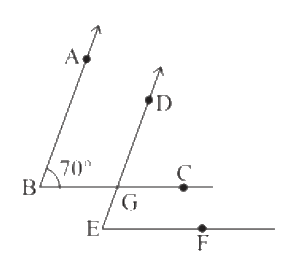### Solution

Reasoning:

Let’s visually model this problem. There is one operation that can be done. According to this model, the resultant value of corresponding angle will be equal to $$\angle DGC.$$

Now, it’s a matter of finding measure of $$\angle DGC.$$

Steps:

(i) Solve for $$\angle DGC$$

Given $$AB || DG$$ and $$BC$$ is transversal

Also,

$$\angle ABC = 70^\circ\text{ (Given)}$$

Since,

$$\angle ABC = \angle DGC\text{(Corresponding angles)}$$

Therefore$$, \angle DGC = 70^\circ→(1)$$

Reasoning:

Let’s visually model this problem . There is one operation that can be done.According to this model, the resultant value of corresponding angle will be equal to $$\angle DGC.$$ Now, it’s a matter of finding measure of $$\angle DEF.$$

Steps:

(ii) Solve for $$\angle DEF$$

Given $$BC || EF$$ and $$DE$$ is transversal

Also, $$\angle DGC = 70^\circ \text{(from 1)}$$

Since,

$$\angle DGC = \angle DEF \text{(Corresponding angles)}$$

Therefore$$, \angle DEF = 70^\circ$$

## Chapter 5 Ex.5.2 Question 6

In the given figures below, decide whether $$l$$  is parallel to $$m.$$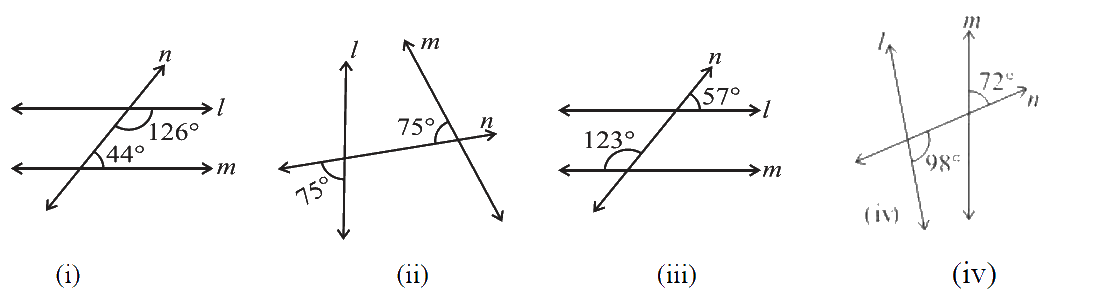### Solution

(i) Reasoning:

Let’s visually model this problem. There is one operation that can be done check whether interior angles are supplementary or not. According to this model, the result sum of $$126^\circ + 44^\circ$$ is $$170^\circ.$$ Now, it’s a matter of finding $$l$$ is parallel to $$m$$ or not

Steps:

(i) $$126^\circ + 44^\circ = 170^\circ$$

As the sum of interior angles on the same side of transversal $$n$$ is not $$180^\circ.$$

Therefore, $$l$$ is not parallel to $$m.$$

(ii) Reasoning:

Let’s visually model this problem. There are two operations that can be done in a sequence. First find the value of $$x$$ and then check it is equal to its corresponding angle or not. According to this model, the resultant value of $$x$$ is not equal to its corresponding angle. Now, it’s a matter of finding $$l$$ is parallel to $$m$$ or not

(ii) Steps:

\begin{align}\angle x + 75^\circ &= 180^\circ \text{(Linear pair)}\\\angle x &= 180^\circ - 75^\circ\\\angle x &= 105^\circ\end{align}

For $$l$$ and $$m$$ to be parallel measure of their corresponding angles should be equal but here the measure of $$\angle x$$ is $$105^\circ$$ and its corresponding angle is $$75^\circ.$$

Therefore, the lines $$l$$ and $$m$$ are not parallel.

(iii) Reasoning:

Let’s visually model this problem. There are two operations that can be done in a sequence. First find the value of $$x$$ and then check it is equal to its corresponding angle or not. According to this model, the resultant value of $$x$$ is not equal to its corresponding angle. Now, it’s a matter of finding $$l$$ is parallel to $$m$$ or not

(iii) Steps:

\begin{align}\angle y&=57^\circ \begin{bmatrix}\text{Vertically opposite}\\\text {angles}\end{bmatrix}\\\angle x + 123^\circ &= 180^\circ\text{ (Linear pair)}\\\angle x& = 180^\circ - 123^\circ\\\angle x &= 57^\circ\end{align}

Here, the measure of corresponding angles are equal i.e $$57^\circ.$$

Therefore, lines l and m are parallel to each other.

(iv) Reasoning:

Let’s visually model this problem. There are two operations that can be done in a sequence. First find the value of $$x$$ by using linear pairand then check it is equal to its corresponding angle or not. According to this model, the resultant value of $$x$$ is not equal to its corresponding angle. Now, it’s a matter of finding $$l$$ is parallel to $$m$$ or not

(iv) Steps:

\begin{align}\angle x + 98^\circ &= 180^\circ \text{(Linear pair)}\\\angle x &= 180^\circ - 98^\circ\\\angle x &= 82^\circ\end{align}

For $$l$$ and $$m$$ to be parallel measure of their corresponding angles should be equal but here the measure of corresponding angles are $$82^\circ$$ and $$72^\circ$$ whichn are not equal.

Therefore, $$l$$ and $$m$$ are not parallel to each other.

Related Sections
Related Sections
Instant doubt clearing with Cuemath Advanced Math Program